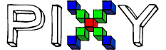# Pixy first version else commands not working properly (İf there are no blocks)

While I did not encounter such a problem in the second version, I am facing such a problem in this version.
I am building a robot that will move when an object is present and not move when it is not.
When the object is present, the robot follows. It stops in the designated area. If there is no object, it stops completely. If there is an object again, it will follow it.
Ok, we can follow the object and stop it wherever we want. However, when we lift the object, the robot starts to turn in other directions. It doesn’t stop.
However, when I type the stop commands, the robot does not move at all.
Of course I’m using the else command while writing this.
When I look at the data on the serial port screen of the Arduino, when the object is defined, it enters the desired block and starts receiving the data. But the robot does not move at all.
motion robot(0,0); When I delete it, the robot starts working.
Actually it doesn’t want to run this very simple code.
I did not understand what you want to do. It’s frustrating that it doesn’t execute such an easy command. I didn’t know what to do and wanted to consult you. Can you help me ??
#include <SPI.h>
#include <Pixy.h>

// This is the main Pixy object
Pixy pixy;

// ENA IN1 IN2 IN3 IN4 ENB
int myPins  = {2, 3, 4, 5, 6, 7};
int b=0;
int cx, cy , c_height, c_width;
void setup()
{
Serial.begin(115200);
Serial.print(“Starting…\n”);
for (int i=0; i<6; i++){
pinMode(myPins[i], OUTPUT);
}
pixy.init();
}

void loop()
{
pixyCheck();

``````if(b==1)
{
cx = pixy.blocks.x;
Serial.println(b);
cy = pixy.blocks.y;
c_height = pixy.blocks.height;
c_width = pixy.blocks.width;

if(cx>180 && cx<320)
{
moverobot(255,-200);
return loop();
}
if(cx>0 && cx<140)
{
moverobot(-255,200);
return loop();
}
if(cx>=140 && cx<=180)
{
if(c_width>60){
moverobot(0,0);
return loop();
}
else {
moverobot(150,150);
return loop();
}
}
}
else                     //It works when I delete these lines.
moverobot(0,0);  //It works when I delete these lines.
``````

}
void pixyCheck()
{
uint16_t blocks;
// grab blocks
blocks=pixy.getBlocks();
// If there are detect blocks, print them!
if (blocks)
{
b=1;

``````for (int i=0; i<blocks; i++)
{
Serial.print("  block ");
Serial.print(i);
Serial.print(": ");
pixy.blocks[i].print();
}
``````

}
else
b=0;
}

void moverobot(int leftSpeed, int rightSpeed)
{
if(leftSpeed ==0 && rightSpeed==0){
analogWrite(myPins, 0);
analogWrite(myPins, 0);
}
else if (leftSpeed > 0 && rightSpeed<0){
digitalWrite(myPins, 0);
digitalWrite(myPins, 1);
analogWrite(myPins, abs(leftSpeed));
analogWrite(myPins, abs(rightSpeed));
digitalWrite(myPins, 1);
digitalWrite(myPins, 0);
analogWrite(myPins, abs(leftSpeed));
analogWrite(myPins, abs(rightSpeed));
}
else if ( rightSpeed >0 && leftSpeed<0){
digitalWrite(myPins, 1);
digitalWrite(myPins, 0);
analogWrite(myPins, abs(leftSpeed));
analogWrite(myPins, abs(rightSpeed));
digitalWrite(myPins, 0);
digitalWrite(myPins, 1);
analogWrite(myPins, abs(leftSpeed));
analogWrite(myPins, abs(rightSpeed));
}
else if ( rightSpeed >0 && leftSpeed>0){
digitalWrite(myPins, 0);
digitalWrite(myPins, 1);
analogWrite(myPins, abs(leftSpeed));
analogWrite(myPins, abs(rightSpeed));
digitalWrite(myPins, 0);
digitalWrite(myPins, 1);
analogWrite(myPins, abs(leftSpeed));
analogWrite(myPins, abs(rightSpeed));
}
}

Is there anyone to help me ??
@edge @freed

Hello,
There’s a difference between the original Pixy’s API and Pixy2. The original API will repeatedly return the same data if you call getBlocks multiple times within the same frame period. The Pixy2 API will block and wait for new data to come in if you call getBlocks more than once within the same frame period.

In order to guarantee that you’re getting new data from Pixy1’s API, you need to wait a frame period (20ms) between calls to getBlocks().

It’s difficult to tell what might be going wrong with your code, but this difference between the API’s can result in different behavior.

Edward

Oh yes. Putting a pause in between worked for me. Thanks.@edge @freed

1 Like